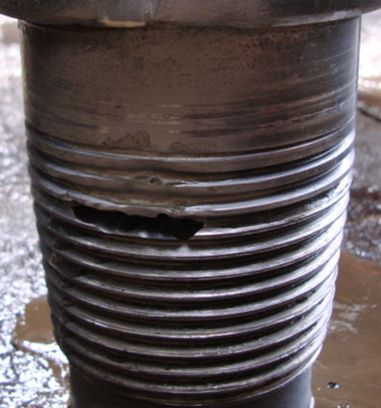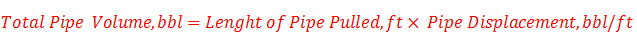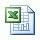## Depth of Washout PipeWashout in drill string can cause big problem later such as parted drill string. When we see stand pipe pressure decrease without changing any parameters as flow rate, mud properties, etc, you may need to consider following items before you decide to pull out of hole for washout.

1. Check surface line: You may need to close stand pipe valves or IBOP and then pressure up to see leaking in the surface. If you see pressure drop, you can fix the surface problem. Anyway you still need to test system again.

2. Check drillstring: You may pump the same flow rate and see how your MWD tool down hole response. If y MWD tool response gets weaker signal so it means that you have washout somewhere above MWD tool. If not, you may have washout below that such as bit, mud motor, etc.You may consider finding washout depth by using two following formulas listed below:

Method 1: The concept of this method is to pump plugging material to plug the wash out. We will count how many strokes pump till pump pressure increases then we can calculate back where the washout is by applying internal capacity concept and pump output concept.

Depth of washout in ft= (strokes pumped till seeing pressure increase x pump output in bbl/stk) ÷ drill pipe capacity in bbl/ft

Determine washout depth from following information:

Internal drill pipe capacity = 0.00742 bbl/ft

Pump output = 0.0855 bbl/stk

Pressure increase was noticed after 400 strokes.

Depth of washout, ft = 400 stk x 0.0855 bbl/stk ÷ 0.00742 bbl/ft

Depth of washout = 4609 ft

Method 2: The concept of this method is to pump material that can be easily observed from drill pipe pass through wash out into annulus and over the surface. We can calculate the depth of washout bases on the combination volume of internal drill pipe volume and annulus volume.

Note: The materials can be easily observed when it comes across the shakers are as follows: carbide, corn starch, glass beads, bright colored paint, etc.

Depth of washout in ft = (strokes pumped till observed material on surface x pump output in bbl/stk) ÷ (drill pipe capacity in bbl/ft + annular capacity in bbl/ft)

Determine depth of washout from following information:

Internal drill pipe capacity = 0.00742 bbl/ft

Pump output = 0.0855 bbl/stk

Annulus capacity = 0.0455 bbl/ft

The material pumped down the drill pipe was noticed coming over the shaker after 2500 strokes.

Depth of washout, ft = (2500 x 0.0855) ÷ (0.00742+0.0455)

Depth of washout = 4039 ft

If you want to subtract volume from bell nipple to shale shaker, you can subtract the volume out of total volume pumped. Therefore the formula will be

Depth of washout, ft = (strokes pumped till observed material on surface x pump output in bbl/stk – volume (bbl) from bell nipple to shale shaker) ÷ (drill pipe capacity in bbl/ft + annular capacity in bbl/ft)

Example: Internal drill pipe capacity capacity = 0.00742 bbl/ft

Pump output = 0.0855 bbl/stk

Annulus capacity = 0.0455 bbl/ft

The material pumped down the drill pipe was noticed coming over the shaker after 2500 strokes.

Volume from bell nipple to shale shaker = 10 bbl

Depth of washout in ft = (2500 x 0.0855 – 10) ÷ (0.00742+0.0455)

Depth of washout = 3850 ft

ANYWAY PLEASE REMEMBER. If you know that your wash out is down hole, practically, we need to pull out of hole ASAP after we determine washout situation. The more you pump, more washout will be occurred.

Please find the excel sheet for calculating depth of washout

Ref books: Lapeyrouse, N.J., 2002. Formulas and calculations for drilling, production and workover, Boston: Gulf Professional publishing.

Bourgoyne, A.J.T., Chenevert , M.E. & Millheim, K.K., 1986. SPE Textbook Series, Volume 2: Applied Drilling Engineering, Society of Petroleum Engineers.

Mitchell, R.F., Miska, S. & Aadny, B.S., 2011. Fundamentals of drilling engineering, Richardson, TX: Society of Petroleum Engineer

## Drill pipe pulled to lose hydrostatic pressureYou previously learn about hydrostatic pressure lose due to pulling out of hole . This post will use the same concept but we will determine how many feet of drill pipe pulled to lose certain amount of hydrostatic pressure in well bore.

The calculations below have 2 cases of pulling out of hole, pull dry and pull wet. They are different in calculation because amount of drilling fluid out of hole is different. Please follow and understand each case of calculation.

#1: How many feet of pipe pulled DRY to lose certain amount of hydrostatic pressure

Feet = (hydrostatic pressure loss in psi x (casing cap in bbl/ft – pipe displacement in bbl/ft)) ÷ (mud weight in ppg x 0.052 x pipe displacement in bbl/ft)

Example: Determine the FEET of dry drill pipe that must be pulled to lose the overbalance using the following data:

Hydrostatic pressure loss = 200 psi
Casing capacity = 0.0873 bbl/ft
Pipe displacement = 0.01876 bbl/ft
Mud weight = 12.0 ppg
Ft = 200 psi x (0.0873 – 0.01876) ÷ (12.0 ppg x 0.052 x 0.01876)
Ft = 1171 ft
You need to pull 1171 ft of dry pipe to lose 200 psi hydrostatic pressure.

#2: How many feet of pipe pulled WET to lose certain amount of hydrostatic pressure

Feet = hydrostatic pressure loss in psi x (casing capacity in bbl/ft – drill pipe capacity in bbl/ft – drill pipe displacement in bbl/ft) ÷ {mud wt in ppg x 0.052 x (pipe displacement in bbl/ft + (% of volume in drill pipe out of hole ÷ 100) x pipe capacity in bbl/ft)}

Example: Determine the feet of WET pipe that must be pulled to lose the overbalance using the following data:

% of volume in drill pipe out of hole = 100
Hydrostatic pressure loss = 200 psi
Casing capacity = 0.0873 bbl/ft
Drill pipe capacity = 0.01876 bbl/ft
Drill pipe displacement = 0.0055 bbl/ft
Mud weight = 12.0 ppg

Feet = 200 psi x (0.0873 – 0.01876 – 0.0055 bbl/ft) ÷ {12.0 ppg x 0.052 x (0.0055 + (100÷100) x 0.01876 bbl/ft)}
Feet = 832.9 ft
You need to pull 833 ft of wet pipe to lose 200 psi hydrostatic pressure.

Please find how many feet of drill pipe pulled to lose certain amount of hydrostatic pressure in well bore.

Ref books: Lapeyrouse, N.J., 2002. Formulas and calculations for drilling, production and workover, Boston: Gulf Professional publishing.

Bourgoyne, A.J.T., Chenevert , M.E. & Millheim, K.K., 1986. SPE Textbook Series, Volume 2: Applied Drilling Engineering, Society of Petroleum Engineers.

Mitchell, R.F., Miska, S. & Aadny, B.S., 2011. Fundamentals of drilling engineering, Richardson, TX: Society of Petroleum Engineers.

## Hydrostatic Pressure (HP) Decreases When POOHWhen pulling out of hole, volume of steel will be out of hole and mud volume will replace the steel volume.  If we don’t fill hole, hydrostatic pressure will decrease. There are 2 cases of pulling pipe which are pull dry and pull wet. Each condition is different in calculation concept because mud volume to displace pipe volume is different.

This topic shows you how to calculate hydrostatic pressure loss for both cases of pulling pipe, pull dry and pull wet. Moreover, there is the Excel sheet for calculating pressure decrease due to pulling out of hole.

Case#1: When pulling DRY pipe

When pulling dry, we will consider volume of steel out of hole only.

Step 1: Determine Total Pipe VolumeStep 2: Determine Hydrostatic Pressure DecreaseExample: Determine the hydrostatic pressure decrease when pulling DRY pipe out of the hole:

Number of stands pulled = 10
Pipe displacement = 0.0055 bbl/ft
Average length per stand = 91 ft
Casing capacity = 0.0873 bbl/ft
Mud weight = 12.0 ppg

Step 1: Determine of pipe displacement in Barrels = 10 stands x 91 ft/std x 0.0055 bbl/ft displaced
Barrels displaced = 5.01 bbl
Step 2: Determine HP, psi decrease = 5.01 barrels x 0.052 x 12.0 ppg ÷ (0.0873 bbl/ft – 0.0055 bbl/ft)
Hydrostatic pressure decrease = 38.2 psi

Case#2: When pulling WET pipe

When pulling wet, we will consider volume of steel out of hole and volume of mud in drillpipe as well. Therefore, pulling wet will decrease hydrostatic more than pulling dry pipe.

Step 1: Barrels displaced = number of stands pulled per stand in ft
x average length x {pipe disp inbbl/ft + {(% volume in drill pipe out of hole ÷ 100) x pipe cap in bbl/ft)}

Step 2: Determine hydrostatic pressure in psi decrease = barrels displaced x 0.052 x mud weight, ppg ÷ ((casing capacity in bbl/ft) – (Pipe disp in bbl/ft + pipe cap in bbl/ft))

Example: Determine the hydrostatic pressure decrease when pulling WET pipe out of the
hole:

% of volume in drill pipe out of hole = 100
Number of stands pulled = 10
Pipe displacement = 0.0055 bbl/ft
Average length per stand = 91 ft
Pipe capacity = 0.01876 bbl/ft
Mud weight = 12.0 ppg
Casing capacity = 0.0873 bbl/ft

Step 1: Barrels displaced = 10 stands x 91 ft/std x {(.0055 bbl/ft + (100 ÷ 100) x 0.01876 bbl/ft)}
Barrels displaced = 22.08 bbl

Step 2: hydrostatic pressure in psi decrease = 22.0766 barrels x 0.052 x 12.0 ppg ÷ ((0.0873 bbl/ft) – (0.0055 bbl/ft + 0.01876 bbl/ft))
HP decrease, psi = 218.52 psi

Ref books: Lapeyrouse, N.J., 2002. Formulas and calculations for drilling, production and workover, Boston: Gulf Professional publishing.

Bourgoyne, A.J.T., Chenevert , M.E. & Millheim, K.K., 1986. SPE Textbook Series, Volume 2: Applied Drilling Engineering, Society of Petroleum Engineers.

Mitchell, R.F., Miska, S. & Aadny, B.S., 2011. Fundamentals of drilling engineering, Richardson, TX: Society of Petroleum Engineers.

## Amount of cuttings produced per foot of hole and total solid generatedAfter learning about capacity calculation, we can apply the capacity calculation to determine how much barrels of cutting produced per foot of hole drilled and total solid generated in pounds.

Please read and understand the following formulas:

Use formula#1 and #2 for calculating amount of cutting generated per feet drilled.

## Formula#1 for BARRELS of cuttings drilled per foot of hole drilled:

Barrels of cutting per foot drilled = Dh2 x (1 – % porosity) ÷1029.4

Where: Dh is hole diameter in inch.

Example: Determine barrels of cuttings drilled for one foot of 6-1/8 inch hole with 25% (0.25) porosity:

Barrels/footage drilled = 6.1252 x (1 – 0.25) ÷1029.4
Barrels/footage drilled = 0.02733 bbl/footage drilled

## Formula#2 for CUBIC FEET of cuttings drilled per foot of hole drilled:

Cubic feet of cutting per foot drilled = Dh2 x 0.7854 x (1 – % porosity) ÷144

Where: Dh is hole diameter in inch.

Example: Determine barrels of cuttings drilled for one foot of 6-1/8 inch hole with 25% (0.25) porosity

Cubic feet/footage drilled = 6.1252 x 0.7854 x (1 – 0.25) ÷144
Cubic feet/footage drilled = 0.153462 cu ft/footage drilled

Moreover, you also apply sample density and volume relationship to determine total solids generated. Use the following formula to calculate total solid generated.

Wcg = 350 x Ch x L x (l – porosity) x Cutting density

Where;

Wcg = solids generated in pounds
Ch = capacity of hole in bbl/ft
L = footage drilled in ft
Cutting density = cutting density in gm/cc

Example: Determine the total pounds of solids generated in drilling 100 ft of  6-1/8 inch hole (0.03644 bbl/ft).

Density of cuttings = 2.20 gm/cc.

Porosity = 25%:
Wcg = 350 x 0.03644 x 100 x (1 – 0.25) x 2.2
Wcg = 2104.41 poundsPlease find the excel sheet how to calculate how much cuttings drilled per foot of hole drilled and total solids generated

Ref books: Lapeyrouse, N.J., 2002. Formulas and calculations for drilling, production and workover, Boston: Gulf Professional publishing.

Bourgoyne, A.J.T., Chenevert , M.E. & Millheim, K.K., 1986. SPE Textbook Series, Volume 2: Applied Drilling Engineering, Society of Petroleum Engineers.

Mitchell, R.F., Miska, S. & Aadny, B.S., 2011. Fundamentals of drilling engineering, Richardson, TX: Society of Petroleum Engineers.